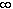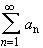#Interactive Real Analysis

Next | Previous | Glossary | Map

## 4.2. Convergence Tests

### Integral Test

Suppose that f(x) is positive, continuous, decreasing function on the interval [N,). Let a n = f(n). Thenconverges if and only ifconverges
Note that this test is much different from all the others. We have not yet formally introduced the concept of an Integral - or even of a continuous function - so that we can not prove this test here. However, for completeness it is included as a test that is sometimes useful to apply.
Next | Previous | Glossary | Map